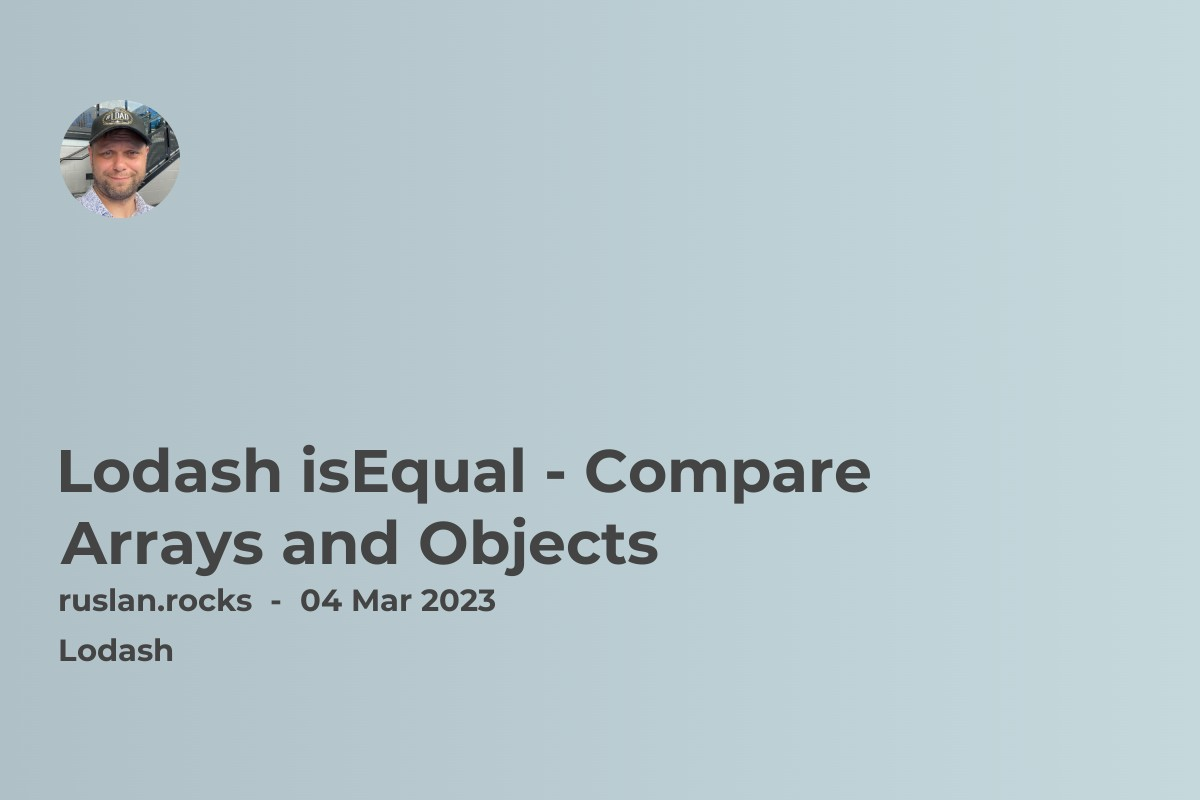# Lodash isEqual - Compare Arrays and ObjectsWhen working with arrays, comparing them is often necessary to check for equality. However, comparing arrays in JavaScript can be tricky because arrays are objects, and two arrays are only equal if they have the same reference in memory. This is where the Lodash library comes in handy with its `isEqual` method.

## #Lodash isEqual method

The `isEqual` method in Lodash is a powerful tool that allows you to compare arrays, objects, and other data types for strict equality. The method takes two arguments and returns a Boolean value indicating whether the two arguments are equal or not.

Here's how to use `isEqual` to compare two arrays:

``````const _ = require('lodash');

const arr1 = [1, 2, 3];

const arr2 = [1, 2, 3];

const arr3 = [1, 2, 4];

console.log(_.isEqual(arr1, arr2)); // true

console.log(_.isEqual(arr1, arr3)); // false
``````

This example has three arrays: `arr1`, `arr2`, and `arr3`. We use the `isEqual` method to compare `arr1` and `arr2`, which should return true since they have the same values at each index. We then compare `arr1` and `arr3`, which should return false because they differ at the third index.

It's important to note that `isEqual` recursively compares the values of each property in an object or element in an array. This means that if an array or object contains other arrays or objects, the method will also compare their values.

Here's an example of how `isEqual` handles nested arrays:

``````const _ = require('lodash');

const arr1 = [[1, 2], [3, 4]];

const arr2 = [[1, 2], [3, 4]];

const arr3 = [[1, 2], [4, 3]];

console.log(_.isEqual(arr1, arr2)); // true

console.log(_.isEqual(arr1, arr3)); // false
``````

In this example, we have two nested arrays: `arr1` and `arr2`. They have the same values at each index, so `isEqual`returns true. We then compare `arr1` and `arr3`, which have different values at the second nested array's second index. Thus, .isEqual()` returns false.

## #How isEqual method works?

``````function isEqual(value, other) {

if (value === other) {

return true;

}

if (

value == null ||

other == null ||

!isObjectLike(value) ||

!isObjectLike(other)

) {

return value !== value && other !== other;

}

return baseIsEqual(value, other, bitmaskEqual, customizer, stack);

}
``````

The `isEqual` function checks if the two input values are strictly equal (`value === other`). If they are, it returns `true`. If either value is `null` or not an object-like value (i.e., it's not an object or an array), it checks if either value is `NaN` using the `value !== value` and `other !== other` comparison. If either value is `NaN`, it returns `true` if both values are `NaN`.

If both values are object-like, the function calls the `baseIsEqual` function with five arguments: `value`, `other`, `bitmaskEqual`, `customizer`, and `stack`. The `baseIsEqual` function performs a deep comparison of the two values and returns a Boolean indicating whether they are equal or not.

The `bitmaskEqual` argument is a bitmask that specifies the types of comparisons to perform (e.g., strict equality, non-strict equality, etc.). The `customizer` argument is a function that allows you to customize the comparison process. The `stack` argument is an array that keeps track of the objects being compared to avoid circular references.

Overall, the `isEqual` function in Lodash is a complex and powerful tool for comparing values in JavaScript.

## #Lodash isEqualWith method

Sure! The `isEqualWith` method in Lodash is similar to the `isEqual` method but allows you to customize the comparison process by providing a custom comparison function. Here's how to use `isEqualWith` to compare arrays:

``````const _ = require("lodash");

const arr1 = [1, 2, 3];

const arr2 = [1, 2, 3];

const arr3 = [1, 2, 4];

function customizer(value1, value2) {

if (Array.isArray(value1) && Array.isArray(value2)) {

return _.isEqualWith(value1, value2, customizer);

}

}

console.log(_.isEqualWith(arr1, arr2, customizer)); // true

console.log(_.isEqualWith(arr1, arr3, customizer)); // false
``````

In this example, we define a customizer function that checks if the two values being compared are arrays. If they are, the function recursively calls `isEqualWith` with the two arrays and the customizer function as arguments. This allows us to perform a deep comparison of the arrays.

We then use `isEqualWith` to compare `arr1` and `arr2`, which should return true since they have the same values at each index. We also compare `arr1` and `arr3`, which should return false because they differ at the third index.

The `isEqualWith` method is useful when you need to compare values in a non-standard way. For example, you might want to compare two arrays element by element but ignore the order of the elements. In this case, you could define a custom comparison function that sorts the elements of each array before comparing them.

Here's an example of how to use `isEqualWith` to compare arrays while ignoring the order of the elements:

``````const _ = require("lodash");

const arr1 = [1, 2, 3];

const arr2 = [3, 2, 1];

function customizer(value1, value2) {

if (Array.isArray(value1) && Array.isArray(value2)) {

value1.sort();

value2.sort();

return _.isEqualWith(value1, value2, customizer);

}

}

console.log(_.isEqualWith(arr1, arr2, customizer)); // true
``````

In this example, we define a customizer function that sorts the values of each array before comparing them. This allows us to compare the arrays while ignoring the order of the elements. We then use `isEqualWith` to compare `arr1` and `arr2`, which should return true because their values are equal after sorting.

The `isEqualWith` method in Lodash is a powerful tool for comparing values in a customizable way. It can be used to compare arrays element-by-element or to ignore certain aspects of the comparison process.

In conclusion, the `isEqual` method in the Lodash library is a powerful tool for comparing arrays and other data types for strict equality. Its ability to recursively compare nested arrays and objects makes it an essential tool for any JavaScript developer.

## Related articlesWritten by author: Ruslan Osipov